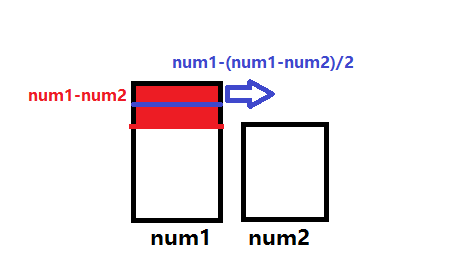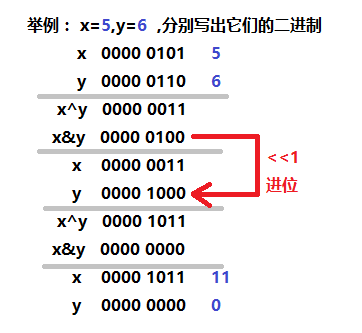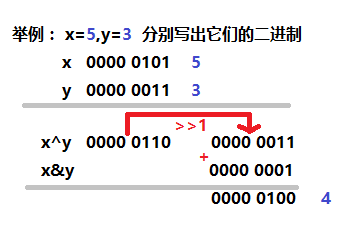# 不使用（a + b） / 2这种方式，求两个数的平均值

##### 我对题目的第一种理解：

(a=b)/2这种方式求平均数时，假如两个数过大的话极易发生溢出现象，通过其他的计算方式避免这种情况。#include<stdio.h>
#include<stdlib.h>
int main()
{
int num1 = 0;
int num2 = 0;
scanf("%d%d", &num1, &num2);
int avg = num1 - (num1 - num2) / 2;
printf("%d", avg);
system("pause");
return 0;
}

##### 我对题目的第二种理解：

①第一步：用add函数 实现加法（代码见下方）②第二步：用avg函数 实现求平均值（代码见下方）③第三步：在主函数中调用avg函数 实现求平均值

#include <stdio.h>
#include <stdlib.h>
int add(int x, int y)//①用来实现加法的函数
{
int tmp = 0;
do
{
tmp = x^y;
y = x&y;
x = tmp;
y <<= 1;//进位
} while (y != 0);
return x;
}

int avg(int x,int y)//②用来求平均值的函数
{
return add(((x^y) >> 1), x&y);
}

int main()//主函数
{
int a = 0;
int b = 0;
printf("Input two number:");
scanf("%d%d",&a,&b);
printf("%d\n",avg(a,b));

system("pause");
return 0;
}